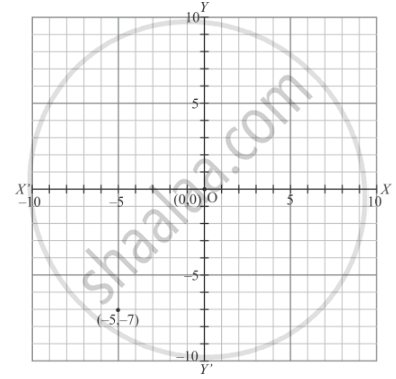# Plot the Following Point on the Graph Paper: (−5, −7) - Mathematics

Plot the following point on the graph paper:

(−5, −7)

#### Solution

The following point is given below.

(−5, −7)

Let X ' OX and  Y' OY be the coordinate axes.

Here for the given point the abscissa is -5 units and ordinate is -7 units.The point is in the third quadrant. So it will look like as shown in the following figure.Concept: Plotting a Point in the Plane If Its Coordinates Are Given.
Is there an error in this question or solution?

#### APPEARS IN

RD Sharma Mathematics for Class 9
Chapter 8 Co-ordinate Geometry
Exercise 8.1 | Q 1.03 | Page 6

Share